University Physics Volume 2

# Problems

### Problems

24.

A 50-turn coil has a diameter of 15 cm. The coil is placed in a spatially uniform magnetic field of magnitude 0.50 T so that the face of the coil and the magnetic field are perpendicular. Find the magnitude of the emf induced in the coil if the magnetic field is reduced to zero uniformly in (a) 0.10 s, (b) 1.0 s, and (c) 60 s.

25.

Repeat your calculations of the preceding problem’s time of 0.1 s with the plane of the coil making an angle of (a) $30°,30°,$ (b) $60°,60°,$ and (c) $90°90°$ with the magnetic field.

26.

A square loop whose sides are 6.0-cm long is made with copper wire of radius 1.0 mm. If a magnetic field perpendicular to the loop is changing at a rate of 5.0 mT/s, what is the current in the loop?

27.

The magnetic field through a circular loop of radius 10.0 cm varies with time as shown below. The field is perpendicular to the loop. Plot the magnitude of the induced emf in the loop as a function of time.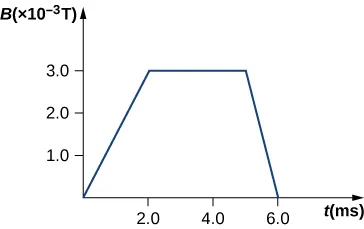28.

The accompanying figure shows a single-turn rectangular coil that has a resistance of $2.0Ω.2.0Ω.$ The magnetic field at all points inside the coil varies according to $B=B0e−αt,B=B0e−αt,$ where $B0=0.25TB0=0.25T$ and $α=200Hz.α=200Hz.$ What is the current induced in the coil at (a) $t=0.001st=0.001s$, (b) 0.002 s, (c) 2.0 s?29.

How would the answers to the preceding problem change if the coil consisted of 20 closely spaced turns?

30.

A long solenoid with $n=10n=10$ turns per centimeter has a cross-sectional area of $5.0cm25.0cm2$ and carries a current of 0.25 A. A coil with five turns encircles the solenoid. When the current through the solenoid is turned off, it decreases to zero in 0.050 s. What is the average emf induced in the coil?

31.

A rectangular wire loop with length a and width b lies in the xy-plane, as shown below. Within the loop there is a time-dependent magnetic field given by $B→(t)=C((xcosωt)i^+(ysinωt)k^)B→(t)=C((xcosωt)i^+(ysinωt)k^)$, with $B→(t)B→(t)$ in tesla. Determine the emf induced in the loop as a function of time.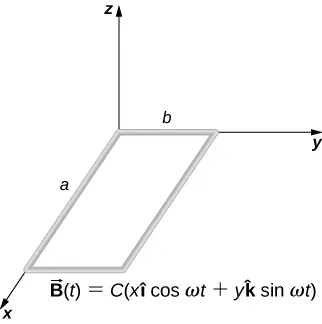32.

The magnetic field perpendicular to a single wire loop of diameter 10.0 cm decreases from 0.50 T to zero. The wire is made of copper and has a diameter of 2.0 mm. How much charge moves through the wire while the field is changing?

### 13.2Lenz's Law

33.

A single-turn circular loop of wire of radius 50 mm lies in a plane perpendicular to a spatially uniform magnetic field. During a 0.10-s time interval, the magnitude of the field increases uniformly from 200 to 300 mT. (a) Determine the emf induced in the loop. (b) If the magnetic field is directed out of the page, what is the direction of the current induced in the loop?

34.

When a magnetic field is first turned on, the flux through a 20-turn loop varies with time according to $Φm=5.0t2−2.0t,Φm=5.0t2−2.0t,$ where $ΦmΦm$ is in milliwebers, t is in seconds, and the loop is in the plane of the page with the unit normal pointing outward. (a) What is the emf induced in the loop as a function of time? What is the direction of the induced current at (b) t = 0, (c) 0.10, (d) 1.0, and (e) 2.0 s?

35.

The magnetic flux through the loop shown in the accompanying figure varies with time according to $Φm=2.00e−3tsin(120πt),Φm=2.00e−3tsin(120πt),$ where $ΦmΦm$ is in webers. What are the direction and magnitude of the current through the $5.00-Ω5.00-Ω$ resistor at (a) $t=0t=0$; (b) $t=2.17×10−2s,t=2.17×10−2s,$ and (c) $t=3.00s?t=3.00s?$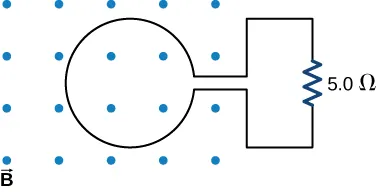36.

Use Lenz’s law to determine the direction of induced current in each case.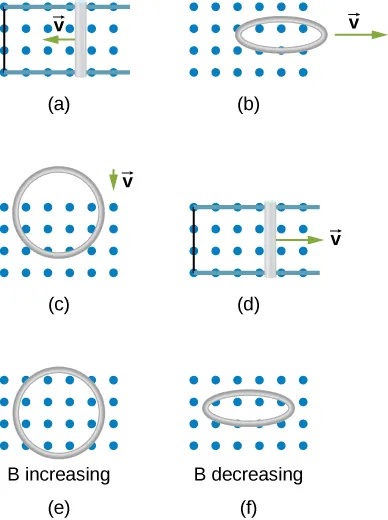### 13.3Motional Emf

37.

An automobile with a radio antenna 1.0 m long travels at 100.0 km/h in a location where the Earth’s horizontal magnetic field is $5.5×10−5T.5.5×10−5T.$ What is the maximum possible emf induced in the antenna due to this motion?

38.

The rectangular loop of N turns shown below moves to the right with a constant velocity $v→v→$ while leaving the poles of a large electromagnet. (a) Assuming that the magnetic field is uniform between the pole faces and negligible elsewhere, determine the induced emf in the loop. (b) What is the source of work that produces this emf?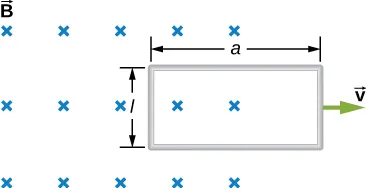39.

Suppose the magnetic field of the preceding problem oscillates with time according to $B=B0sinωt.B=B0sinωt.$ What then is the emf induced in the loop when its trailing side is a distance d from the right edge of the magnetic field region?

40.

A coil of 1000 turns encloses an area of $25cm225cm2$. It is rotated in 0.010 s from a position where its plane is perpendicular to Earth’s magnetic field to one where its plane is parallel to the field. If the strength of the field is $6.0×10−5T,6.0×10−5T,$ what is the average emf induced in the coil?

41.

In the circuit shown in the accompanying figure, the rod slides along the conducting rails at a constant velocity $v→.v→.$ The velocity is in the same plane as the rails and directed at an angle $θθ$ to them. A uniform magnetic field $B→B→$ is directed out of the page. What is the emf induced in the rod?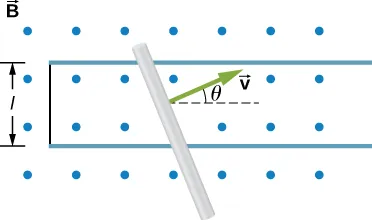42.

The rod shown in the accompanying figure is moving through a uniform magnetic field of strength $B=0.50TB=0.50T$ with a constant velocity of magnitude $v=8.0m/s.v=8.0m/s.$ What is the potential difference between the ends of the rod? Which end of the rod is at a higher potential?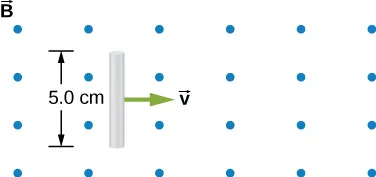43.

A 25-cm rod moves at 5.0 m/s in a plane perpendicular to a magnetic field of strength 0.25 T. The rod, velocity vector, and magnetic field vector are mutually perpendicular, as indicated in the accompanying figure. Calculate (a) the magnetic force on an electron in the rod, (b) the electric field in the rod, and (c) the potential difference between the ends of the rod. (d) What is the speed of the rod if the potential difference is 1.0 V?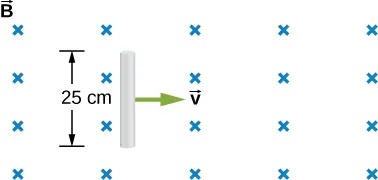44.

In the accompanying figure, the rails, connecting end piece, and rod all have a resistance per unit length of $2.0Ω/cm.2.0Ω/cm.$ The rod moves to the left at $v=3.0m/s.v=3.0m/s.$ If $B=0.75TB=0.75T$ everywhere in the region, what is the current in the circuit (a) when $a=8.0cm?a=8.0cm?$ (b) when $a=5.0cm?a=5.0cm?$ Specify also the sense of the current flow.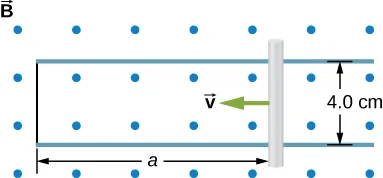45.

The rod shown below moves to the right on essentially zero-resistance rails at a speed of $v=3.0m/s.v=3.0m/s.$ If $B=0.75TB=0.75T$ everywhere in the region, what is the current through the $5.0-Ω5.0-Ω$ resistor? Does the current circulate clockwise or counterclockwise?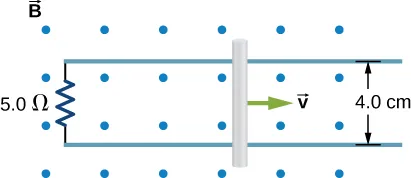46.

Shown below is a conducting rod that slides along metal rails. The apparatus is in a uniform magnetic field of strength 0.25 T, which is directly into the page. The rod is pulled to the right at a constant speed of 5.0 m/s by a force $F→.F→.$ The only significant resistance in the circuit comes from the $2.0-Ω2.0-Ω$ resistor shown. (a) What is the emf induced in the circuit? (b) What is the induced current? Does it circulate clockwise or counter clockwise? (c) What is the magnitude of $F→F→$? (d) What are the power output of $F→F→$ and the power dissipated in the resistor?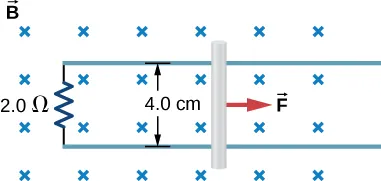### 13.4Induced Electric Fields

47.

Calculate the induced electric field in a 50-turn coil with a diameter of 15 cm that is placed in a spatially uniform magnetic field of magnitude 0.50 T so that the face of the coil and the magnetic field are perpendicular. This magnetic field is reduced to zero in 0.10 seconds. Assume that the magnetic field is cylindrically symmetric with respect to the central axis of the coil.

48.

The magnetic field through a circular loop of radius 10.0 cm varies with time as shown in the accompanying figure. The field is perpendicular to the loop. Assuming cylindrical symmetry with respect to the central axis of the loop, plot the induced electric field in the loop as a function of time.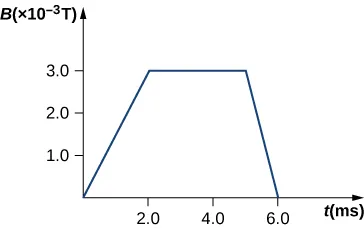49.

The current I through a long solenoid with n turns per meter and radius R is changing with time as given by dI/dt. Calculate the induced electric field as a function of distance r from the central axis of the solenoid.

50.

Calculate the electric field induced both inside and outside the solenoid of the preceding problem if $I=I0sinωt.I=I0sinωt.$

51.

Over a region of radius R, there is a spatially uniform magnetic field $B→.B→.$ (See below.) At $t=0t=0$, $B=1.0T,B=1.0T,$ after which it decreases at a constant rate to zero in 30 s. (a) What is the electric field in the regions where $r≤Rr≤R$ and $r≥Rr≥R$ during that 30-s interval? (b) Assume that $R=10.0cmR=10.0cm$. How much work is done by the electric field on a proton that is carried once clock wise around a circular path of radius 5.0 cm? (c) How much work is done by the electric field on a proton that is carried once counterclockwise around a circular path of any radius $r≥Rr≥R$? (d) At the instant when $B=0.50TB=0.50T$, a proton enters the magnetic field at A, moving a velocity $v→v→$ $(v=5.0×106m/s)(v=5.0×106m/s)$ as shown. What are the electric and magnetic forces on the proton at that instant?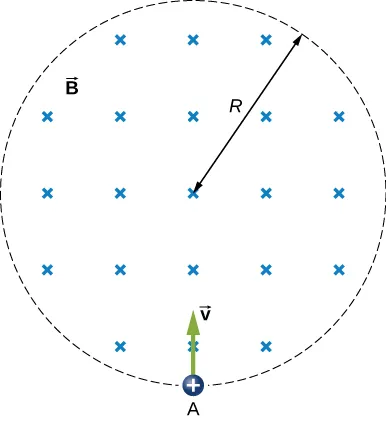52.

The magnetic field at all points within the cylindrical region whose cross-section is indicated in the accompanying figure starts at 1.0 T and decreases uniformly to zero in 20 s. What is the electric field (both magnitude and direction) as a function of r, the distance from the geometric center of the region?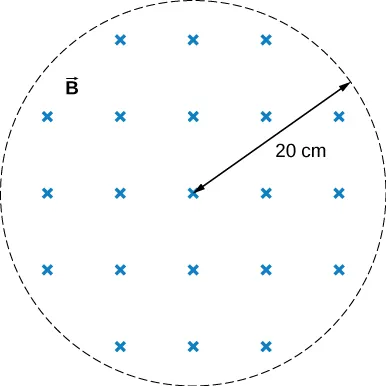53.

The current in a long solenoid with 20 turns per centimeter of radius 3 cm is varied with time at a rate of 2 A/s. A circular loop of wire of radius 5 cm and resistance $2Ω2Ω$ surrounds the solenoid. Find the electrical current induced in the loop.

54.

The current in a long solenoid of radius 3 cm and 20 turns/cm is varied with time at a rate of 2 A/s. Find the electric field at a distance of 4 cm from the center of the solenoid.

### 13.6Electric Generators and Back Emf

55.

Design a current loop that, when rotated in a uniform magnetic field of strength 0.10 T, will produce an emf $ε=ε0sinωt,ε=ε0sinωt,$ where $ε0=110Vε0=110V$ and $ω=120πrad/s.ω=120πrad/s.$

56.

A flat, square coil of 20 turns that has sides of length 15.0 cm is rotating in a magnetic field of strength 0.050 T. If the maximum emf produced in the coil is 30.0 mV, what is the angular velocity of the coil?

57.

A 50-turn rectangular coil with dimensions $0.15m×0.40m0.15m×0.40m$ rotates in a uniform magnetic field of magnitude 0.75 T at 3600 rev/min. (a) Determine the emf induced in the coil as a function of time. (b) If the coil is connected to a $1000-Ω1000-Ω$ resistor, what is the power as a function of time required to keep the coil turning at 3600 rpm? (c) Answer part (b) if the coil is connected to a 2000-$ΩΩ$ resistor.

58.

The square armature coil of an alternating current generator has 200 turns and is 20.0 cm on side. When it rotates at 3600 rpm, its peak output voltage is 120 V. (a) What is the frequency of the output voltage? (b) What is the strength of the magnetic field in which the coil is turning?

59.

A flip coil is a relatively simple device used to measure a magnetic field. It consists of a circular coil of N turns wound with fine conducting wire. The coil is attached to a ballistic galvanometer, a device that measures the total charge that passes through it. The coil is placed in a magnetic field $B→B→$ such that its face is perpendicular to the field. It is then flipped through $180°,180°,$ and the total charge Q that flows through the galvanometer is measured. (a) If the total resistance of the coil and galvanometer is R, what is the relationship between B and Q? Because the coil is very small, you can assume that $B→B→$ is uniform over it. (b) How can you determine whether or not the magnetic field is perpendicular to the face of the coil?

60.

The flip coil of the preceding problem has a radius of 3.0 cm and is wound with 40 turns of copper wire. The total resistance of the coil and ballistic galvanometer is $0.20Ω.0.20Ω.$ When the coil is flipped through $180°180°$ in a magnetic field $B→,B→,$ a change of 0.090 C flows through the ballistic galvanometer. (a) Assuming that $B→B→$ and the face of the coil are initially perpendicular, what is the magnetic field? (b) If the coil is flipped through $90°,90°,$ what is the reading of the galvanometer?

61.

A 120-V, series-wound motor has a field resistance of 80 $ΩΩ$ and an armature resistance of 10 $ΩΩ$. When it is operating at full speed, a back emf of 75 V is generated. (a) What is the initial current drawn by the motor? When the motor is operating at full speed, where are (b) the current drawn by the motor, (c) the power output of the source, (d) the power output of the motor, and (e) the power dissipated in the two resistances?

62.

A small series-wound dc motor is operated from a 12-V car battery. Under a normal load, the motor draws 4.0 A, and when the armature is clamped so that it cannot turn, the motor draws 24 A. What is the back emf when the motor is operating normally?

Order a print copy

As an Amazon Associate we earn from qualifying purchases.